In this article we refer to “set functions” as functions that operate on sets. The three set functions available in DAX are: UNION, INTERSECT, and EXCEPT. Their behavior is very intuitive:

• UNION performs the union of two or more tables.
• INTERSECT performs the set intersection between two tables.
• EXCEPT removes the rows of the second argument from the first one.

These functions take two or more tables as parameters and return a table. They prove useful not only to write DAX queries; a developer can also use these functions to prepare complex filters when implementing measures.

In their most common use the set functions maintain the data lineage, which is of paramount importance when preparing filters. If the lineage is lost, you can use TREATAS to either restore the lineage or force a new one.

We start with the basics of the set functions, followed by insights about the data lineage.

## UNION

UNION takes two or more tables and returns a table with all the rows of all the tables received as parameters. The structure of the result is the same as the structure of the source tables, and duplicates – if present – are kept. If you need to remove duplicates, you can use DISTINCT over UNION.

To implement an example with UNION, we use two variables; each contains a table with the column Day of Week, and each row represents one weekday. We numbered the weekdays starting from Sunday. Consequently, 1 stands for Sunday, 2 for Monday and 7 for Saturday:

```EVALUATE
VAR SunMon =
CALCULATETABLE (
VALUES ( 'Date'[Day of Week] ),
'Date'[Day of Week Number] IN { 1, 2 }
)
RETURN
SunMon
```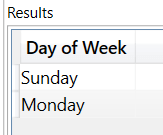```EVALUATE
VAR MonTue =
CALCULATETABLE (
VALUES ( 'Date'[Day of Week] ),
'Date'[Day of Week Number] IN { 2, 3 }
)
RETURN
MonTue
```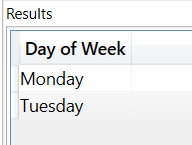Each of these variables contains two rows. In the following example, we use UNION over the two tables. The result is a table containing all the rows of the source tables, including duplicates:

```EVALUATE
VAR SunMon =
CALCULATETABLE (
VALUES ( 'Date'[Day of Week] ),
'Date'[Day of Week Number] IN { 1, 2 }
)
VAR MonTue =
CALCULATETABLE (
VALUES ( 'Date'[Day of Week] ),
'Date'[Day of Week Number] IN { 2, 3 }
)
VAR UnionResult = UNION ( SunMon, MonTue )
RETURN
UnionResult
```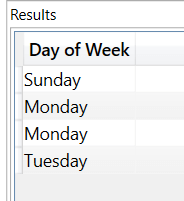As we can see, the result contains all the rows from both tables and the duplicate row Monday is not removed.

DISTINCT proves useful to remove duplicates:

```EVALUATE
VAR SunMon =
CALCULATETABLE (
VALUES ( 'Date'[Day of Week] ),
'Date'[Day of Week Number] IN { 1, 2 }
)
VAR MonTue =
CALCULATETABLE (
VALUES ( 'Date'[Day of Week] ),
'Date'[Day of Week Number] IN { 2, 3 }
)
VAR UnionResult = UNION( SunMon, MonTue )
VAR DistinctResult = DISTINCT( UnionResult )
RETURN
DistinctResult
```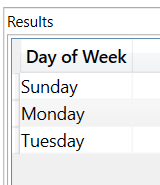## INTERSECT

INTERSECT accepts two tables as arguments. It returns all the rows in the first argument that are also present in the second argument, and it retains any duplicates present in the first argument. The order of the parameters matters: duplicates are kept only if present in the first argument.

In the following example, we use INTERSECT on the same days of the week temporary tables that were used for the previous examples. The result contains only Monday, because it is the only weekday in common between the two tables:

```EVALUATE
VAR SunMon =
CALCULATETABLE (
VALUES ( 'Date'[Day of Week] ),
'Date'[Day of Week Number] IN { 1, 2 }
)
VAR MonTue =
CALCULATETABLE (
VALUES ( 'Date'[Day of Week] ),
'Date'[Day of Week Number] IN { 2, 3 }
)
VAR IntersectResult = INTERSECT( SunMon, MonTue )
RETURN
IntersectResult
```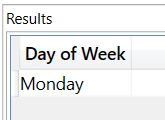To experiment with duplicates, we add the SunMonMonWed variable, that contains the union of SunMon and MonWed. This table contains Monday twice; therefore, it holds a duplicate:

```EVALUATE
VAR SunMon =
CALCULATETABLE (
VALUES ( 'Date'[Day of Week] ),
'Date'[Day of Week Number] IN { 1, 2 }
)
VAR MonWed =
CALCULATETABLE (
VALUES ( 'Date'[Day of Week] ),
'Date'[Day of Week Number] IN { 2, 4 }
)
VAR SunMonMonWed = UNION ( SunMon, MonWed )
RETURN
SunMonMonWed
```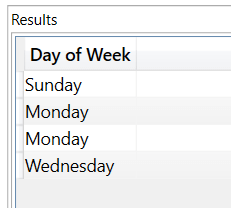In the following example, we use INTERSECT over SunMonMonWed as the first parameter and MonTue as the second parameter. The result contains only Monday, but duplicated; indeed, MonTue contains Monday and none of the other days in SunMonMonWed:

```EVALUATE
VAR SunMon =
CALCULATETABLE (
VALUES ( 'Date'[Day of Week] ),
'Date'[Day of Week Number] IN { 1, 2 }
)
VAR MonWed =
CALCULATETABLE (
VALUES ( 'Date'[Day of Week] ),
'Date'[Day of Week Number] IN { 2, 4 }
)
VAR SunMonMonWed = UNION ( SunMon, MonWed )
VAR MonTue =
CALCULATETABLE (
VALUES ( 'Date'[Day of Week] ),
'Date'[Day of Week Number] IN { 2, 3 }
)
VAR IntersectResult = INTERSECT ( SunMonMonWed, MonTue )
RETURN
IntersectResult
```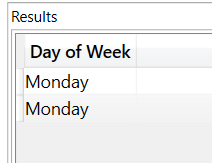Changing the order of the parameters changes the result. Because Monday only appears once in the MonTue variable – that we now use as the first argument – the result contains Monday only once:

```EVALUATE
VAR SunMon =
CALCULATETABLE (
VALUES ( 'Date'[Day of Week] ),
'Date'[Day of Week Number] IN { 1, 2 }
)
VAR MonWed =
CALCULATETABLE (
VALUES ( 'Date'[Day of Week] ),
'Date'[Day of Week Number] IN { 2, 4 }
)
VAR SunMonMonWed = UNION ( SunMon, MonWed )
VAR MonTue =
CALCULATETABLE (
VALUES ( 'Date'[Day of Week] ),
'Date'[Day of Week Number] IN { 2, 3 }
)
VAR IntersectResult = INTERSECT( MonTue, SunMonMonWed )
RETURN
IntersectResult
```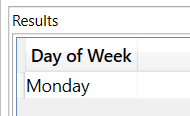## EXCEPT

The third and last of the set functions is EXCEPT. Except accepts two tables as arguments and it returns all the rows in Table1 that are not present in Table2. When using EXCEPT, the order of the parameters is of paramount importance. Indeed, EXCEPT retains duplicates only if present in the first argument.

As a first example, we use EXCEPT with SunMon as the first parameter and MonTue as the second parameter. The result is a table with only Sunday, because Monday is present in the second parameter and will be removed from the result:

```EVALUATE
VAR SunMon =
CALCULATETABLE (
VALUES ( 'Date'[Day of Week] ),
'Date'[Day of Week Number] IN { 1, 2 }
)
VAR MonTue =
CALCULATETABLE (
VALUES ( 'Date'[Day of Week] ),
'Date'[Day of Week Number] IN { 2, 3 }
)
VAR ExceptResult = EXCEPT( SunMon, MonTue )
RETURN
ExceptResult
```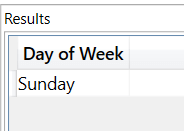In the next example, we change the order of the parameters; we use EXCEPT with MonTue as the first parameter and SunMon as the second parameter. The result contains only Tuesday, because it is the only weekday that is not present in the second parameter:

```EVALUATE
VAR SunMon =
CALCULATETABLE (
VALUES ( 'Date'[Day of Week] ),
'Date'[Day of Week Number] IN { 1, 2 }
)
VAR MonTue =
CALCULATETABLE (
VALUES ( 'Date'[Day of Week] ),
'Date'[Day of Week Number] IN { 2, 3 }
)
VAR ExceptResult = EXCEPT( MonTue, SunMon )
RETURN
ExceptResult
```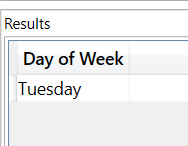## Tables with different column names and data lineage

The arguments of set functions may have both different column names and a different data lineage. These functions match the columns across the tables by their position. When the name of a column in the same position is different, the result uses the name in the first table.

Regarding the data lineage, the behavior depends on the set function: when the data lineages of the arguments are different, UNION loses the data lineage whereas INTERSECT and EXCEPT both maintain the lineage of their first argument.

We can see some of the behaviors of UNION in practice with a few examples.

The first example shows UNION being used with different column names. The following code uses UNION on three different tables with one column that has a different name in each table. The result uses the name of the column in the first table:

```EVALUATE
UNION ( ROW ( "DAX", 1 ), ROW ( "is a", 1 ), ROW ( "Language", 2 ) )
```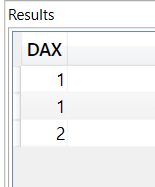The second example shows that the data lineage is maintained when UNION is used over two tables with the same data lineage. To do so, we added a measure evaluation to our first code sample. The result contains a different value in each row, according to the Day of Week column:

```EVALUATE
VAR SunMon =
CALCULATETABLE (
VALUES ( 'Date'[Day of Week] ),
'Date'[Day of Week Number] IN { 1, 2 }
)
VAR MonTue =
CALCULATETABLE (
VALUES ( 'Date'[Day of Week] ),
'Date'[Day of Week Number] IN { 2, 3 }
)
VAR UnionResult = UNION( SunMon, MonTue )
RETURN
UnionResult,
"Sales", [Sales Amount]
)
```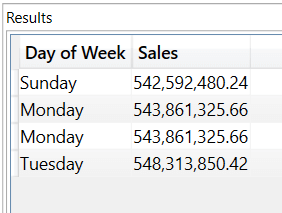Because the data lineage of both tables is the same ‘Date'[Day of Week] column, UNION keeps the data lineage. Monday appears twice because UNION does not remove duplicates.

In the next example, the data lineage is lost when UNION is used over tables with a different data lineage. We replaced MonTue with MyMonTue that contains the same days but without a data lineage. Because the two arguments of UNION have a different data lineage, the result loses the data lineage. Furthermore, the evaluation of the measure produces the same number for all the rows:

```EVALUATE
VAR SunMon =
CALCULATETABLE (
VALUES ( 'Date'[Day of Week] ),
'Date'[Day of Week Number] IN { 1, 2 }
)
VAR MyMonTue = { "Monday", "Tuesday" }
VAR UnionResult = UNION( SunMon, MyMonTue )
RETURN
UnionResult,
"Sales", [Sales Amount]
)
```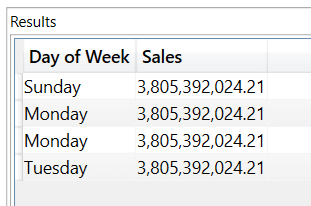If needed, you can restore the data lineage by using TREATAS. To demonstrate this, we created the MyMonTueDataLineage variable that uses TREATAS to restore the data lineage. The result is now the sales amount for each day of the week, because of the restored data lineage:

```EVALUATE
VAR SunMon =
CALCULATETABLE (
VALUES ( 'Date'[Day of Week] ),
'Date'[Day of Week Number] IN { 1, 2 }
)
VAR MyMonTue = { "Monday", "Tuesday" }
VAR MyMonTueDataLineage =
TREATAS ( MyMonTue, 'Date'[Day of Week] )
VAR UnionResult =
UNION ( SunMon, MyMonTueDataLineage )
RETURN
UnionResult,
"Sales", [Sales Amount]
)
```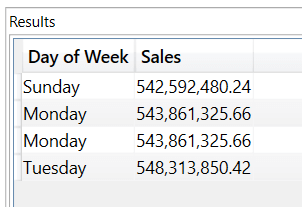## Tables with a different number of columns

None of the set functions accept arguments with a different number of columns.

In the following example, we use EXCEPT over SunMon (two columns) and MonTue (one column). The result is an error:

```EVALUATE
VAR SunMon =
CALCULATETABLE (
SUMMARIZE ('Date', 'Date'[Day of Week], 'Date'[Day of Week Number] ),
'Date'[Day of Week Number] IN { 1, 2 }
)
VAR MonTue =
CALCULATETABLE (
VALUES ( 'Date'[Day of Week] ),
'Date'[Day of Week Number] IN { 2, 3 }
)
VAR ExceptResult = EXCEPT( SunMon, MonTue ) -- ERROR
RETURN
ExceptResult
```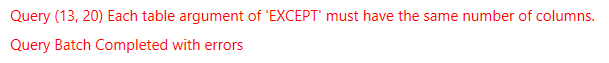## Tables with different column types

What happens if the arguments of a set function have the same number of columns, but the corresponding columns have a different data type? In this case, UNION behaves differently from INTERSECT and EXCEPT. Indeed, UNION converts column types from numeric to string, whereas INTERSECT and EXCEPT do not apply any conversion and return an error instead. On the other hand, conversions between numerical types work for all the set functions.

In the following example, we create two tables, T1 with two columns of type STRING and INTEGER and T2 with the opposite configuration. We then apply UNION which returns a table with two columns, both of type STRING:

```EVALUATE
VAR T1 =
DATATABLE ( "C1", STRING, "C2", INTEGER, { { "A", 1 }, { "B", 2 } } )
VAR T2 =
DATATABLE ( "C3", INTEGER, "C4", STRING, { { 3, "C" }, { 4, "D" } } )
RETURN
UNION ( T1, T2 )
```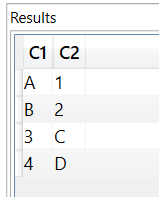The same example with INTERSECT or EXCEPT returns an error:

```EVALUATE
VAR T1 =
DATATABLE ( "C1", STRING, "C2", INTEGER, { { "A", 1 }, { "B", 2 } } )
VAR T2 =
DATATABLE ( "C3", INTEGER, "C4", STRING, { { 3, "C" }, { 4, "D" } } )
RETURN
INTERSECT ( T1, T2 ) – ERROR
```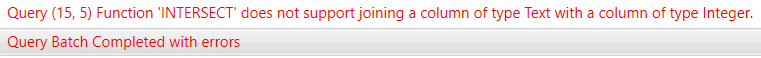This last example shows a working conversion between two different numerical types using INTERSECT. The returned table contains 1, which is the expected intersection between T1 and T2:

```EVALUATE
VAR T1 =
DATATABLE ( "C1", INTEGER, { { 1 }, { 2 } } )
VAR T2 =
DATATABLE ( "C1", DOUBLE, { { 1 }, { 4 } } )
RETURN
INTERSECT ( T1, T2 )
```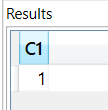## Conclusions

The beauty of set functions is that they are simple to use, and they typically work exactly as you would expect. The most relevant topic when using set functions is the data lineage. By following the rules outlined in this article, you can easily predict whether the data lineage is kept or lost. In case it is lost, you can use TREATAS to restore it.

UNIONReturns the union of the tables whose columns match.

`UNION ( <Table>, <Table> [, <Table> [, … ] ] )`

INTERSECTReturns the rows of left-side table which appear in right-side table.

`INTERSECT ( <LeftTable>, <RightTable> )`

EXCEPTReturns the rows of left-side table which do not appear in right-side table.

`EXCEPT ( <LeftTable>, <RightTable> )`

TREATASTreats the columns of the input table as columns from other tables.For each column, filters out any values that are not present in its respective output column.

`TREATAS ( <Expression>, <ColumnName> [, <ColumnName> [, … ] ] )`

DISTINCTReturns a one column table that contains the distinct (unique) values in a column, for a column argument. Or multiple columns with distinct (unique) combination of values, for a table expression argument.

`DISTINCT ( <ColumnNameOrTableExpr> )`